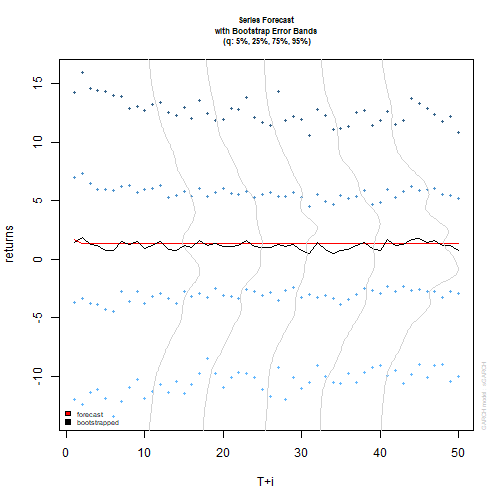# Volatility modelling in R exercises (Part-4)

July 17, 2017
By

Want to share your content on R-bloggers? click here if you have a blog, or here if you don't.This is the fourth part of the series on volatility modelling. For other parts of the series follow the tag volatility.

In this exercise set we will explore GARCH-M and E-GARCH models. We will also use these models to generate rolling window forecasts, bootstrap forecasts and perform simulations.

Answers to the exercises are available here.

Exercise 1
Load the `rugarch` and the `FinTS` packages. Next, load the `m.ibmspln` dataset from the `FinTS` package. This dataset contains monthly excess returns of the S&P500 index and IBM stock from Jan-1926 to Dec-1999 (Ruey Tsay (2005) Analysis of Financial Time Series, 2nd ed. ,Wiley, chapter 3).
Also, load the `forecast` package which we will use for autocorrelation graphs.

Exercise 2
Estimate a GARCH(1,1)-M model for the S&P500 excess returns series. Determine if the effect of volatility on asset returns is significant.

Exercise 3
Excess IBM stock returns are defined as a regular zoo variable. Convert this to a time series variable with correct dates.

Learn more about Model Evaluation in the online course Regression Machine Learning with R. In this course you will learn how to:

• Avoid model over-fitting using cross-validation for optimal parameter selection
• Explore maximum margin methods such as best penalty of error term support vector machines with linear and non-linear kernels.
• And much more

Exercise 4
Plot the absolute and squared excess IBM stock returns along with its ACF and PACF graphs and determine the appropriate model configuration.

Exercise 5
The exponential GARCH model incorporates asymmetric effects for positive and negative asset returns. Estimate an AR(1)-EGARCH(1,1) model for the IBM series.

Exercise 6
Using the results from exercise-5, get rolling window forecasts starting from the 800th observation and refit the model after every three observations.

Exercise 7
Estimate an AR(1)-GARCH(1,1) model for the IBM series and get a bootstrap forecast for the next 50 periods with 500 replications.

Exercise 8
Plot the forecasted returns and sigma with bootstrap error bands.

Exercise 9
We can use Monte-Carlo simulation to get a distribution of the parameter estimates. Using the fitted model from exercise-7, run the simulation for 500 periods for a horizon of 2000 periods.

Exercise 10
Plot the density functions of the parameter estimates.

R-bloggers.com offers daily e-mail updates about R news and tutorials about learning R and many other topics. Click here if you're looking to post or find an R/data-science job.
Want to share your content on R-bloggers? click here if you have a blog, or here if you don't.